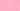Pricing# Analysis of Variance (ANOVA)

How do you compare two or more sets of data? There’s a multitude of options when it comes to statistical analysis. One way to get to the bottom of discrepancies in your testing is with an analysis of variance test.

## What is variance analysis?

Analysis of variance (ANOVA) is a type of test used in statistics to see if there’s a difference between two groups. This tool analyses the difference between planned and actual numbers. To do this, an ANOVA test splits the variability found within any data set into two sections:

• Systematic factors – factors with a statistical influence on the data set

• Random factors – factors with no statistical influence

When running the variance analysis, you can determine the degree of influence the independent variables have on the dependent variable. In other words, an analysis of variance is used to determine whether the results of an experiment are significant or random.

## Types of variances

You could apply this statistical review to many different variables that crop up within the business world. Here are some of the main types of variances to explore:

• Labour variance

• Sales variance

• Budget variance

• Material variance

## What is analysis of variance used for?

In business, an analysis of variance is used to assess any deviations in a company’s financial performance. Plus, it helps management perform an additional control check on operational performance, keeping processes within budget.

The ANOVA test offers a way to analyse the various factors that impact your data set, exploring inconsistencies. An analyst uses these tools to generate additional data that aligns more consistently with regression models. When there’s no significant difference between the two tested groups, this is called a ‘null hypothesis,’ and the ANOVA test’s F-ratio should be close to 1.

## Variance analysis formula and calculations

There’s no single variance analysis formula for all investigations. The variance analysis you perform will depend on the type of variable you’re analysing. Here are some of the main variance analysis formulas:

### Material cost variance formula:

Standard Cost – Actual Cost = (Standard Quantity x Standard Price) – (Actual Quantity X Actual Price)

### Labour variance formula:

Standard Wages – Actual Wages = (Standard Hours x Standard Price) – (Actual Hours x Actual Price)

(Actual Output x Standard Rate) – Actual Fixed Overhead

### Sales variance formula:

(Budgeted Quality x Budgeted Price) – (Actual Quality x Actual Price)

In most cases, analysts will run these formulas using software like Excel. However, it’s also possible to perform an ANOVA test by hand using the following steps:

1. Find the mean for each group that you’re comparing.

2. Calculate the overall mean, or mean of the combined groups.

3. Calculate the within-group variation, or deviation of each score from the group mean.

4. Find the between-group variation, or deviation of each group mean from the overall mean.

5. Find your F-ratio, or ratio of between-group variation to within-group variation.

## One-way analysis of variance vs. two-way analysis of variance

All the variables and formulas mentioned above fall into two primary types of ANOVA tests. Analysts can choose between one-way or two-way tests, referring to the number of independent variables involved. A one-way analysis of variance will look at a single factor on a single variable. A two-way test looks at two independent factors. For example, a business might compare employee productivity based on factors like education and salary grade.

### One-way analysis of variance

The one-way ANOVA is the simplest type of test, comparing means from two independent groups using the F-ratio. According to the null hypothesis, if these two means are equal, the result is significant.

The one-way ANOVA is useful for comparing two groups, and it can tell you if they’re different from one another. However, it has some limitations. It won’t tell you which groups are deviating or different.

### Two-way analysis of variance

While one-way ANOVA compares two groups, a two-way ANOVA compares two independent variables with a measurement variable. Interaction effects between these factors are compared by making multiple observations, with an F-ratio calculated for each hypothesis. Certain assumptions must be made for this type of ANOVA, including equal sample sizes for each group.

## We can help

GoCardless helps you automate payment collection, cutting down on the amount of admin your team needs to deal with when chasing invoices. Find out how GoCardless can help you with ad hoc payments or recurring payments.

GoCardless makes it easy to collect recurring payments